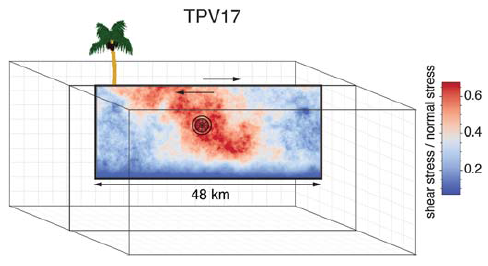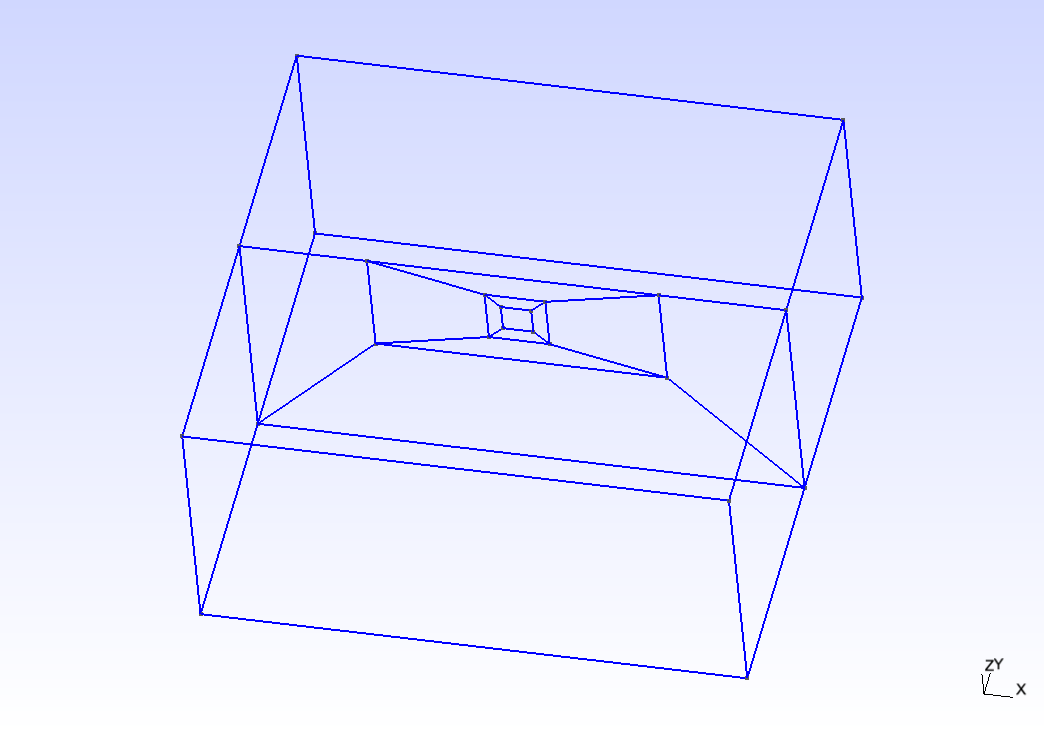# SCEC TPV16/17

TPV16/17 has spontaneous rupture on a vertical, right-lateral, strike-slip fault in a homogeneous half-space with randomly-generated heterogeneous initial stress conditions. The earthquake rupture is artificially nucleated in a circular zone on the fault surface. The rupture then spontaneously propagates outward on the fault surface and encounters heterogeneous stochastic initial stress conditions, some of which prevent it from propagating into certain regions on the fault surface.Diagram of TPV16/17. The fault is 40 km long. Colors indicate the ratio of shear stress to normal stress at locations on the fault surface, at the beginning of the simulation.

## Geometry

The fault is a vertical, planar, right-lateral, strike-slip fault. The fault reaches the Earth’s surface. Rupture is allowed within a rectangular area measuring 48000 m along-strike and 19500 m down-dip. A node which lies exactly on the border of the 48000 m $$\times$$ 19500 m rectangle is considered to be inside the rectangle, and so should be permitted to slip.

The portions of the fault below, to the left of, and to the right of the 48000 m $$\times$$ 19500 m rectangle are a strength barrier, within which the fault is not allowed to rupture.

In the example, a vertical fault is generated with Gmsh in Figure [fig:tpv16mesh]. All the files that are needed for the simulation are provided in https://github.com/SeisSol/Examples/tree/master/tpv16.Fault geometry of TPV16. Planar fault with nucleation size of 200 m.

## Material parameter

Rock properties are taken to be linear elastic throughout the 3D model volume. The problem description can be found at . Table [table:tpv16material] lists all the material parameters.

Parameter

Description

Value

Unit

$$\lambda$$

Lame’s first parameter

3.2044e10

Pa

$$\mu$$

shear module

3.2038e10

Pa

$$\rho$$

density

2670

$$kg/m^{3}$$

$$Q_p$$

P-wave attenuation

69.3

$$Q_s$$

S-wave attenuation

155.9

$$h_{edge}$$

element edge length

200

m

Table: Table of bulk and material parameters in TPV16/17.

## Nucleation parameters

Initial stress (Ts0) is randomly-generated in TPV16/17 (Figure [fig:tpv16ts]).

In TPV16/17, a two-stage nucleation method is used. The first stage is a circular zone of forced rupture which surrounds the hypocenter. Its radius is approximately 1 km (the exact radius is determined as part of the stochastic method that generates the initial stresses). At the hypocenter, the value of then increases with distance from the hypocenter, which creates an expanding circular region of forced rupture. The forced rupture expands at a speed of for 80% of the way, and then for the remaining 20% of the way to the edge of the zone. Outside the zone of forced rupture, is equal to 1.0E9, which means that forced rupture does not occur outside the zone.

The second stage is a circular zone of reduced which surrounds the hypocenter. Its radius is approximately 4 km (the exact radius is determined as part of the stochastic method that generates the initial stresses). In the innermost 10% of the zone, equals 0.04 m. The value of then increases linearly with distance from the hypocenter and reaches its final value of 0.4 m at the edge of the zone. Outside the zone, equals 0.4 m. The effect is to create a circular region of reduced fracture energy surrounding the hypocenter, which helps the rupture to expand during the early part of the simulation.

## Results

The earthquake nucleates and the rupture propagates on the fault surface due to the heterogenous stress ratio on the fault. Figure [fig:tpv16slip] shows the fault slip rate along strike-direction at T=5.5 s.